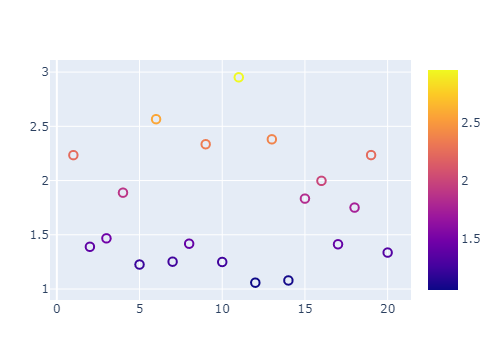# Plots.jl white marker with coloured stroke

Using the GR backend, I would like to have scatter plots where the inside of the markers are white whilst the edge/stroke is the color of the current palette.

The problem is that setting `markercolor=:white` will also make the edge white if `markerstrokecolor=:auto` is set.

MWE:

``````begin
using Plots
gr()
# this will show 100 random points that are white with white stroke
scatter(rand(100), markercolor=:white, markerstrokewidth=1.7, markerstrokecolor=:auto)
end
``````

Thanks in advance for any ideas! (:

It seems that this is impossible, according to this open issue: How to plot unfilled markers? · Issue #2338 · JuliaPlots/Plots.jl · GitHub
With PlotlyJS you can plot such markers, as follows:

``````using PlotlyJS
x= 1:20
y=1 .+2*rand(20)
pl = Plot(scatter(x=x, y=y, mode="markers", marker=attr(color=y,
symbol="circle-open", line_width=2,
size=8.5, showscale=true)), Layout(width=500, height=360,
#template=:plotly_white
))
``````Uncommenting `template=:plotly_white`, the background is removed, as well as the default colorscheme. In the former case it was `:plasma`, while in the latter, `:matter`.

1 Like

setting `markerstrokecolor = :black` works though

1 Like

This is exactly the workaround I have been using. Thanks!

Only downside is that the color of the markers has to be specified on a per-function-call basis.

You can also set

``````default( markerstrokecolor = :black )
``````

Just for completeness, here is a MWE of how to color only the stroke of the markers: whilst keeping their color white:

``````begin
using Plots
x = 0:0.1:2pi
y1 = sin.(x)
y2 = cos.(x)
scatter(x, y1, mc=:white, msc=1)
scatter!(x, y2, mc=:white, msc=2)
end
``````